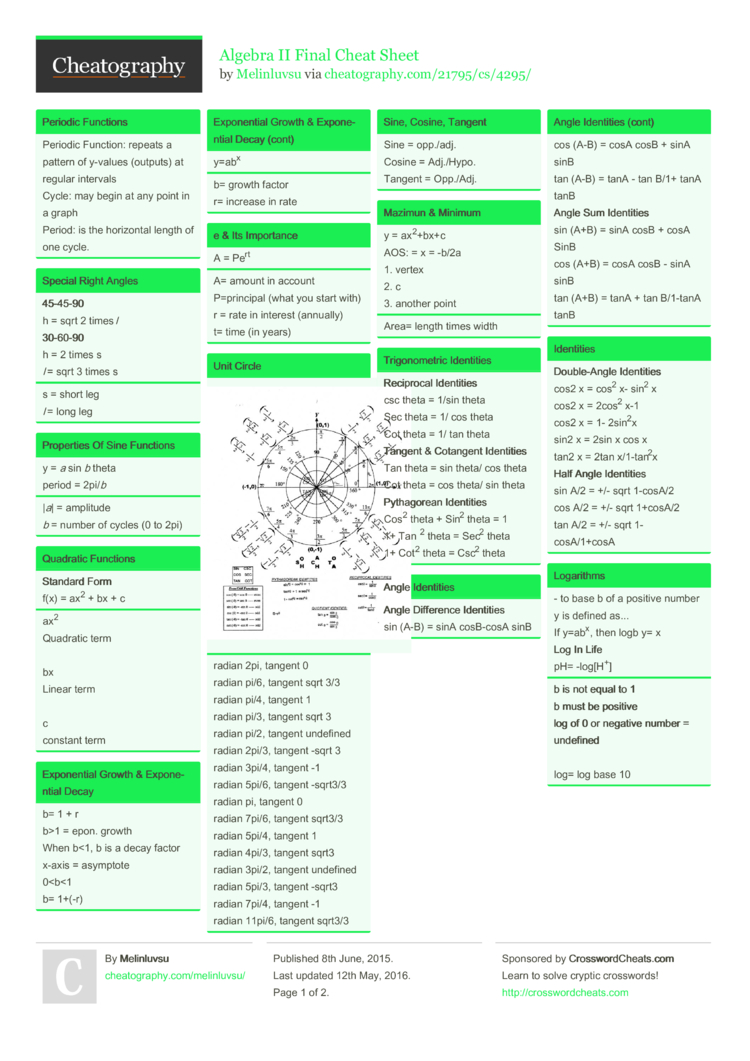# Algebra II Final Cheat Sheet by Melinluvsu

For the use of Algebra II Final

### Periodic Functions

 Periodic Function: repeats a pattern of y-values (outputs) at regular intervals Cycle: may begin at any point in a graph Period: is the horizontal length of one cycle.

### Special Right Angles

 45-4­5-90 h = sqrt 2 times l 30-6­0-90 h = 2 times s l = sqrt 3 times s
s = short leg
l = long leg

### Properties Of Sine Functions

 y = a sin b theta period = 2pi/b
|a| = amplitude
b = number of cycles (0 to 2pi)

 Standard Form f(x) = ax2 + bx + c
ax2

bx
Linear term

c
constant term

### Expone­ntial Growth & Expone­ntial Decay

 b= 1 + r b>1 = epon. growth When b<1, b is a decay factor x-axis = asymptote 0
b= growth factor
r= increase in rate

### e & Its Import­ance

 A = Pert
A= amount in account
r = rate in interest (annually)
t= time (in years)

### Unit Circle### Mazimun & Minimum

 y = ax2+bx+c AOS: = x = -b/2a 1. vertex 2. c 3. another point
Area= length times width

### Trigon­ometric Identities

 Reci­procal Identi­ties csc theta = 1/sin theta Sec theta = 1/ cos theta Cot theta = 1/ tan theta Tangent & Cotangent Identi­ties Tan theta = sin theta/ cos theta Cot theta = cos theta/ sin theta Pyth­agorean Identi­ties Cos2 theta + Sin2 theta = 1 1+ Tan 2 theta = Sec2 theta 1+ Cot2 theta = Csc2 theta

### Angle Identities

 Angle Difference Identi­ties sin (A-B) = sinA cosB-cosA sinB cos (A-B) = cosA cosB + sinA sinB tan (A-B) = tanA - tan B/1+ tanA tanB Angle Sum Identi­ties sin (A+B) = sinA cosB + cosA SinB cos (A+B) = cosA cosB - sinA sinB tan (A+B) = tanA + tan B/1-tanA tanB

### Identities

 Doub­le-­Angle Identi­ties cos2 x = cos2 x- sin2 x cos2 x = 2cos2 x-1 cos2 x = 1- 2sin2x sin2 x = 2sin x cos x tan2 x = 2tan x/1-ta­n2x Half Angle Identi­ties sin A/2 = +/- sqrt 1-cosA/2 cos A/2 = +/- sqrt 1+cosA/2 tan A/2 = +/- sqrt 1-cosA­/1+cosA

### Logarithms

 - to base b of a positive number y is defined as... If y=abx, then logb y= x Log In Life pH= -log[H+]
b is not equal to 1
b must be positive
log of 0 or negative number = undefi­ned

log= log base 10

### Log Are Inverses Of Expone­ntials

 1. Graph expone­netial function 2. Graph y = x 3. Reflect expone­ntial function over y = x (reverse coodin­ates)

### Properties Of Log

 logb MN = logb M+ logb N <--­--p­roduct property logb M/N= logb M - logb N <---- Quotient property logb Mx = x logb M <--­--Power property
WATCH OUT FOR ERRORS
logb a/logb c does not equal logb a/c

logb a times c does not equal logb a times logb c

### Expanding Log

 log2 7b = log2 7 + log 2 b
left to right = expand

right to left = simplify

### Natural Log

 Write 3ln6 - ln8 as a single natural log ln 63/8 ---> ln 216/8 ---> ln 27

### Solving Log Equations

 Pt 1 solve log(3x+1) = 5 3x+1 = 105 3x+1 = 100000 3x = 99,999 x = 33,333 Pt 2 Solve 2log x- log 3 = 2 log(x­2/3)=2 x2/3 = 10^2 x2= 2(100) x=10sqrt3 or 17.32

### Pairs Of Relations are Inverse Of Each Other

 y = x - 7/2 y = 2x+7 y = 3x - 1 y = x +1/3 y = -x + 4 y = -x + 4/-1 y = x + 4/5 y = 5x - 42 Pages
//media.cheatography.com/storage/thumb/melinluvsu_algebra-ii-final.750.jpg

PDF (recommended)